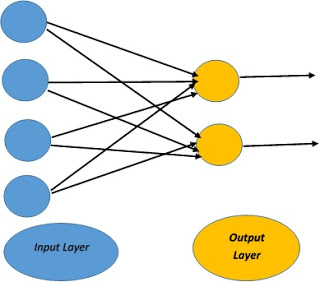/  Deep Learning Interview questions and answers   /  Understanding single layer Perceptron and difference between Single Layer vs Multilayer Perceptron## Understanding single layer Perceptron and difference between Single Layer vs Multilayer Perceptron

Ans: Single layer perceptron is a simple Neural Network which contains only one layer. The single layer computation of perceptron is the calculation of sum of input vector with the value multiplied by corresponding vector weight. The displayed output value will be the input of an activation function.The perceptron consists of 4 parts.

1. Input values or One input layer
2. Weights and Bias
3. Net sum
4. Activation Function

Single-layer Perceptron

Single Layer Perceptron has just two layers of input and output. It only has single layer hence the name single layer perceptron. It does not contain Hidden Layers as that of Multilayer perceptron.Input nodes are connected fully to a node or multiple nodes in the next layer. A node in the next layer takes a weighted sum of all its inputs

Multi-Layer Perceptron (MLP)

A multilayer perceptron is a type of feed-forward artificial neural network that generates a set of outputs from a set of inputs. An MLP is a neural network connecting multiple layers in a directed graph, which means that the signal path through the nodes only goes one way. The MLP network consists of input, output, and hidden layers. Each hidden layer consists of numerous perceptron’s which are called hidden layers or hidden unit.